TitleCollege Algebra
Answer/Discussion to Practice Problems
Tutorial 19: Radical Equations and
Equations Involving Rational Exponents

WTAMU > Virtual Math Lab > College Algebra > Tutorial 19: Radical Equations and Equations Involving Rational ExponentsAnswer/Discussion to 1a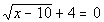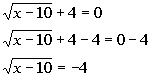*Inverse of add. 4 is sub. 4 *Square root is by itself on one side of eq.

 If you square a square root, it will disappear.  This is what we want to do here so that we can get x out from under the square root and continue to solve for it.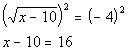*Inverse of taking the sq. root is squaring it

 Step 3: If you still have a radical left, repeat steps 1 and 2.

 No more radicals exist, so we do not have to repeat steps 1 and 2.

 Step 4: Solve the remaining equation.

 In this example, the equation that resulted from squaring both sides turned out to be a linear equation. If you need a review on solving linear equations, feel free to go to Tutorial 14: Linear Equations in One Variable.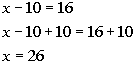*Inverse of sub. 10 is add. 10

 Step 5:  Check for extraneous solutions.

 Let's check to see if x = 26 is an extraneous solution: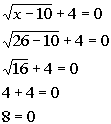*Plugging in 26 for x   *False statement

 Since we got a false statement, x = 26 is an extraneous solution. There is no solution to this radical equation.Answer/Discussion to 1bThe radical in this equation is already isolated.

 If you square a square root, it will disappear.  This is what we want to do here so that we can get y out from under the square root and continue to solve for it.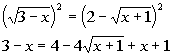*Inverse of taking the sq. root is squaring it *Right side is a binomial squared

 Be careful on this one.  It is VERY TEMPTING to square the right side term by term and get  4 + (x + 1).  However, you need to square it as a side as shown above.  Recall that when you square a binomial you get the first term squared minus twice the product of the two terms plus the second term squared.  If you need a review on squaring a binomial, feel free to go to Tutorial 6: Polynomials.

 Step 3: If you still have a radical left, repeat steps 1 and 2.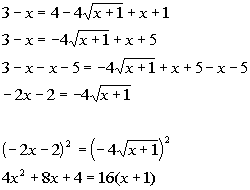*Inverse of add. x and 5 is sub. x and 5   *Square root is by itself on one side of eq.   *Inverse of taking the sq. root is squaring it *Left side is a binomial squared

 Be careful on this one.  It is VERY TEMPTING to square the left side term by term and get  4x squared plus 4.  However, you need to square it as a side as shown above.  Recall that when you square a binomial you get the first term squared plus twice the product of the two terms plus the second term squared.  If you need a review on squaring a binomial, feel free to go to Tutorial 6: Polynomials.

 Step 4: Solve the remaining equation.

 In this example, the equation that resulted from squaring both sides turned out to be a quadratic equation. If you need a review on solving quadratic equations feel free to go to Tutorial 17:  Quadratic Equations.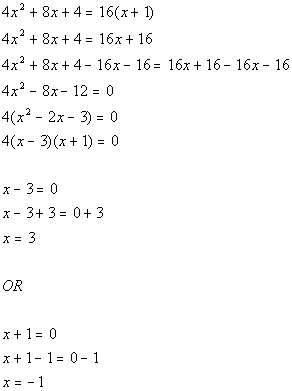*Quad. eq. in standard form *Factor out a GCF of 4 *Factor the trinomial *Use Zero-Product Principle *Set 1st factor = 0 and solve           *Set 2nd factor = 0 and solve

 Step 5:  Check for extraneous solutions.

 Let's check to see if  x = 3 is an extraneous solution: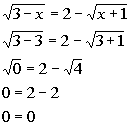*Plugging in 3 for x     *True statement

 Since we got a true statement, x = 3 is a solution.

 Let's check to see if x = -1 is an extraneous solution: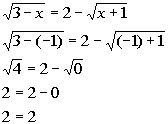*Plugging in -1 for x     *True statement

 Since we got a true statement, x = -1 is a solution. There are two solutions to this radical equation: x = 3 and x = -1.Answer/Discussion to 2a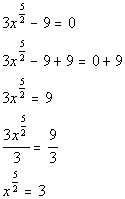*Inverse of sub. 9 is add. 9   *Inverse of mult. by 3 is div. by 3   *rat. exp. expression is by itself on one side of eq.

 If you raise an expression that has a rational exponent to the reciprocal of that rational exponent, the exponent will disappear.   This is what we want to do here so that we can get x out from under the rational exponent and continue to solve for it.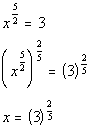*Inverse of taking it to the  5/2 power is taking it to the 2/5 power

 Step 3: Solve the remaining equation.

 In this example, the equation that resulted from raising both sides to the 2/5 power turned out to be a linear equation. Also note that it is already solved for x.  So, we do not have to do anything on this step for this example.

 Step 4:  Check for extraneous solutions.

 Let's check to see if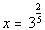is an extraneous solution: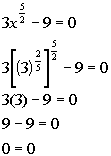*Plugging in 3 to the 2/5 power for x     *True statement

 Since we got a true statement,is a solution. There is one solution to this rational exponent equation:.Answer/Discussion to 2b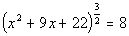The base with the rational exponent is already isolated.

 If you raise an expression that has a rational exponent to the reciprocal of that rational exponent, the exponent will disappear.   This is what we want to do here so that we can get x out from under the rational exponent and continue to solve for it.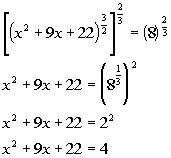*Inverse of taking it to the 3/2 power is taking it to the 2/3 power

 Step 3: Solve the remaining equation.

 In this example, the equation that resulted from squaring both sides turned out to be a quadratic equation. If you need a review on solving quadratic equations, feel free to go to Tutorial 17:  Quadratic Equations.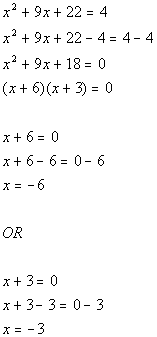*Quad. eq. in standard form *Factor the trinomial *Use Zero-Product Principle *Set 1st factor = 0 and solve           *Set 2nd factor = 0 and solve

 Step 4:  Check for extraneous solutions.

 Let's check to see if x = -6 is an extraneous solution: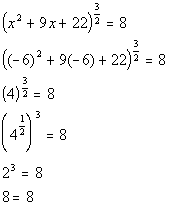*Plugging in -6 for x           *True statement

 Since we got a true statement, x = -6 is a solution.

 Lets check to see if x = -3 is an extraneous solution: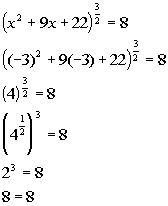*Plugging in -3 for x           *True statement

 Since we got a true statement, x = -3 is a solution. There are two solutions to this rational exponent equation: x = -6 and x = -3.

WTAMU > Virtual Math Lab > College Algebra > Tutorial 19: Radical Equations and Equations Involving Rational Exponents

Last revised on Dec. 16, 2009 by Kim Seward.
All contents copyright (C) 2002 - 2010, WTAMU and Kim Seward. All rights reserved.